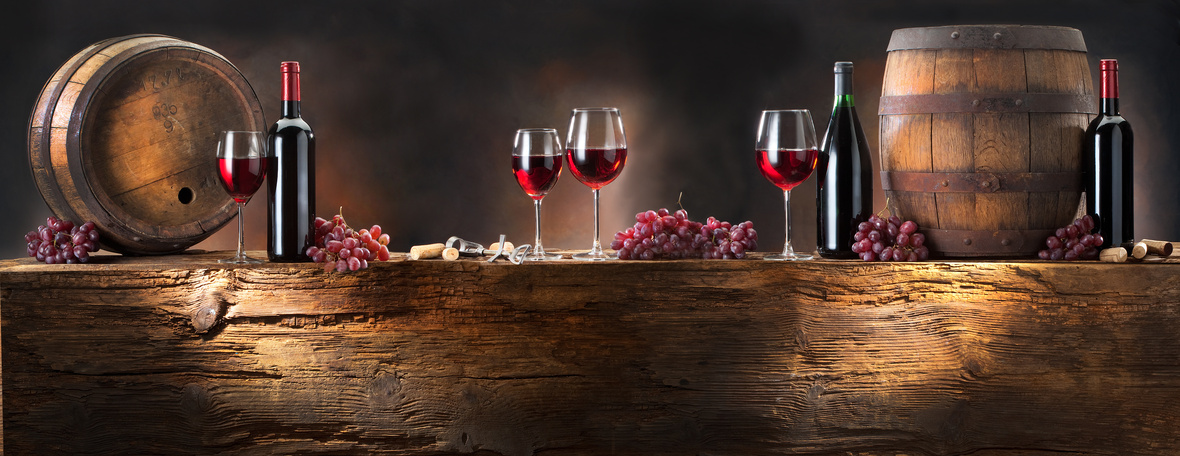# Women's History Month, Spring Refresh and News

 /* styles */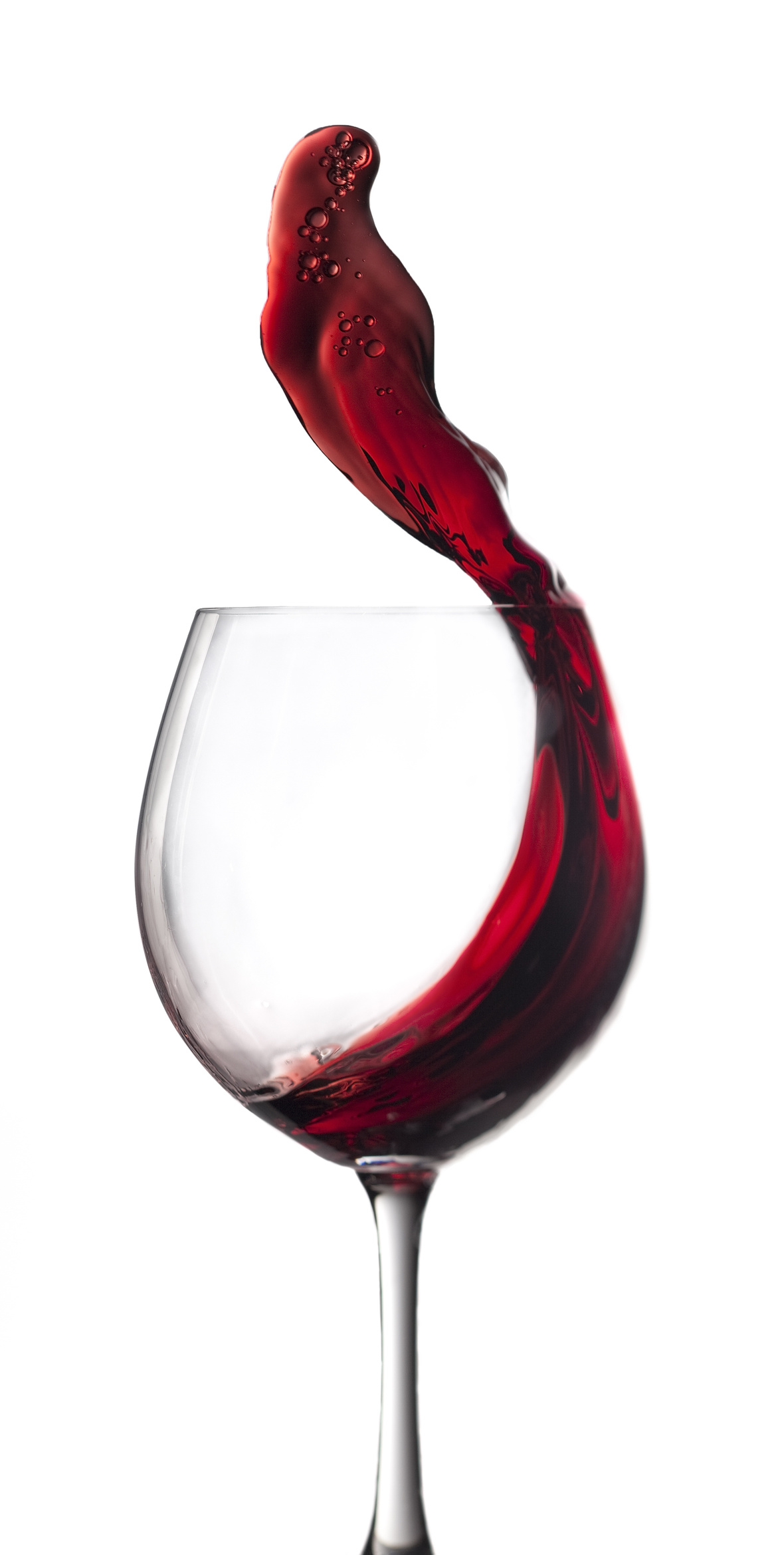Hi Wine & Spirits Lover,

The Shall We Wine team is excited to share our monthly newsletter. Our goal is to introduce you to our favorite selections, producers, distillers, varietals, regions and places to purchase and drink fabulous wine and spirits!

Cheers,

The Shall We Wine Team

 /* styles */

## CHEERS TO WOMENShall We Wine celebrates all women, all day, every day. However, Women's History Month is a special time to focus, reflect and remind ourselves of all the amazing contributions of women to humanity and culture.

Thank you to each woman, breaking barriers and leading the way for change. From challenging inequality and paving the way for future generations of women... you are seen, you are appreciated, you are extraordinary!

Standing ovation for women everywhere. Together, we are unstoppable!

 table div table+table+table+table+table+table+table+table div table{width:100%;padding:0}table div table+table+table+table+table+table+table+table div table img{width:96.23%;padding:0;float:none}table div table+table+table+table+table+table+table+table div table td{width:100%;padding:0 1.88% 18px}/* styles */## VIRTUAL PERFORMANCE FROM SOL COLLECTIVE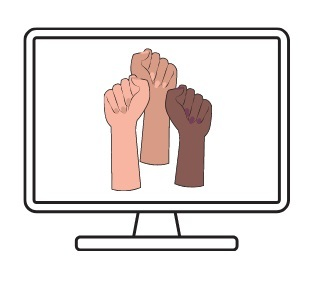SOL Collective will be holding a special production via Zoom!

SOL Collective is a storytelling collective. Founded by Shelley A. Davis, DeeDee Pacheco, and Kara Wright, SOL Collective is a new effort envisioning storytelling as systems change. In a performance arts scene that primarily revolves around competition, folklore, open mic, or cis-male heteronormative audiences, they are seeking to build original work created by and performed by womxn of color.

They were fortunate to receive a grant from Links Hall which allowed them to produce a launch show. Emily Hooper Lansana of Tell Open Stories is the Artistic Director.

When: Monday, March 15, 2021
Time: 7:00 PM- 8:30 PM CST

Please show your support and save the date, share with your networks, and attend the virtual show!

 table div table+table+table+table+table+table+table+table+table+table+table div table{width:100%;padding:0}table div table+table+table+table+table+table+table+table+table+table+table div table img{width:96.23%;padding:0;float:none}table div table+table+table+table+table+table+table+table+table+table+table div table td{width:100%;padding:0 1.88% 18px}/* styles */## LIVE TASTING 3/17/21 WITH ANT MOORE ON IGLIVEJoin us for a live tasting with winemaker Ant Moore. We will be tasting Ant Moore 2020 Estate Sauvignon Blanc, 2020 Estate Pinot Gris and 2020 Signature Rose on Wednesday, March 17 at 6:00 PM CST on IGLive @ShallWeWine. Purchase your bottles and taste along with us!

When: Wednesday, March 17, 2021
Where: @ShallWeWine IG
Time: 6:00 PM- 6:30 PM CST

FEATURED WINES:
2020 Estate Sauvignon Blanc
2020 Estate Pinot Gris
2020 Signature Rose

@HEB – Texas
@central_market – Texas
@WholeFoodsMarket – Illinois
@brooklynwineexchange – New York
@astorwines – New York
@legacylittlerock – Arkansas
@junglejimsmarket – Ohio
@lagrandorange – Phoenix, Arizona, and Florida

 table div table+table+table+table+table+table+table+table+table+table+table+table+table+table div table{width:100%;padding:0}table div table+table+table+table+table+table+table+table+table+table+table+table+table+table div table img{width:96.23%;padding:0;float:none}table div table+table+table+table+table+table+table+table+table+table+table+table+table+table div table td{width:100%;padding:0 1.88% 18px}/* styles */## 2021 WINE EDUCATION SERIES WITH SWW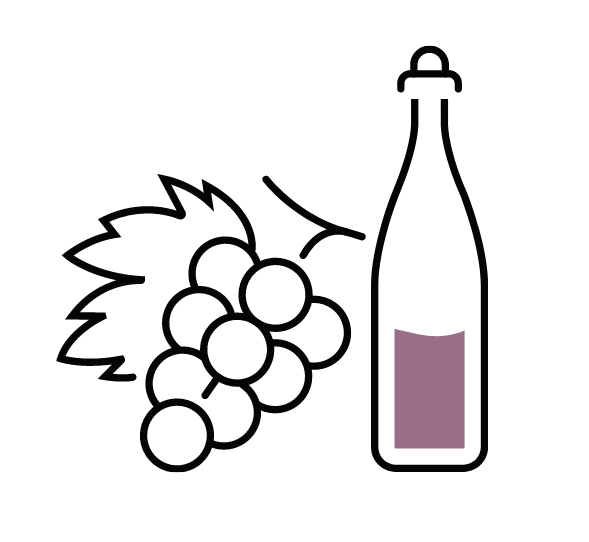Please join Regine T. Rousseau, Founder and CEO for more of the amazing line-up of educational events in 2021!

See the list below, and click to reserve your spot.

Want to level up your wine drinking style without the extra fluff? Shall We Wine is a resource that helps translate complex terminology into language that individuals of every level of wine & spirits proficiency can appreciate… all while having fun!

The goal of these classes is to empower you to find the wines that fit your palate, the foods you enjoy and your mood. If you enjoy wine and are looking to deepen your knowledge of wine styles, grapes and regions, these are the classes for you!

 table div table+table+table+table+table+table+table+table+table+table+table+table+table+table+table+table+table div table{width:100%;padding:0}table div table+table+table+table+table+table+table+table+table+table+table+table+table+table+table+table+table div table img{width:96.23%;padding:0;float:none}table div table+table+table+table+table+table+table+table+table+table+table+table+table+table+table+table+table div table td{width:100%;padding:0 1.88% 18px}/* styles */table div table+table+table+table+table+table+table+table+table+table+table+table+table+table+table+table+table+table+table div table{width:100%;padding:0}table div table+table+table+table+table+table+table+table+table+table+table+table+table+table+table+table+table+table+table div table img{width:96.23%;padding:0;float:none}table div table+table+table+table+table+table+table+table+table+table+table+table+table+table+table+table+table+table+table div table td{width:100%;padding:0 1.88% 18px}/* styles */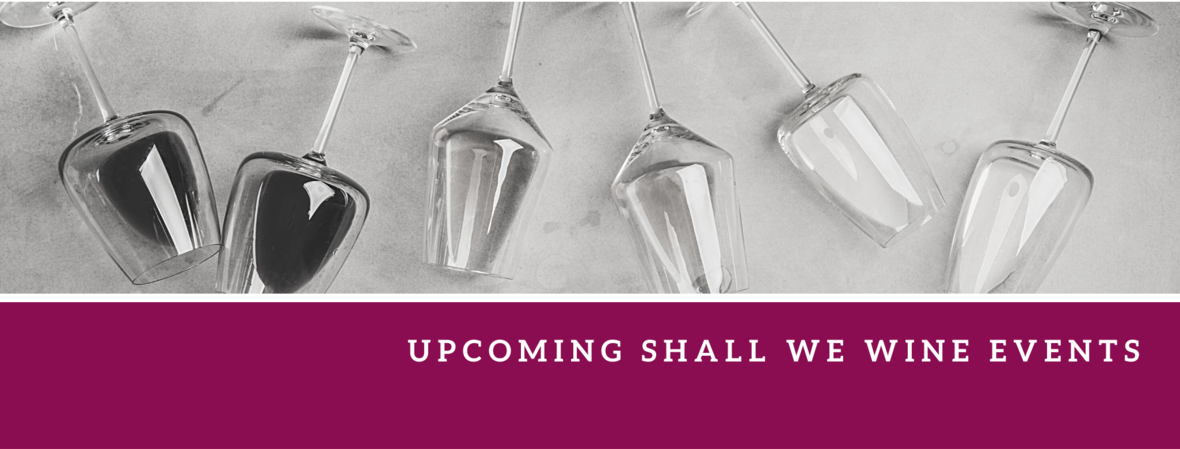/* styles */ Check out our complete events calendar to see what your next pour should be!table div table+table+table+table+table+table+table+table+table+table+table+table+table+table+table+table+table+table+table+table+table+table+table+table+table div table{width:100%;padding:0}table div table+table+table+table+table+table+table+table+table+table+table+table+table+table+table+table+table+table+table+table+table+table+table+table+table div table img{width:96.23%;padding:0;float:none}table div table+table+table+table+table+table+table+table+table+table+table+table+table+table+table+table+table+table+table+table+table+table+table+table+table div table td{width:100%;padding:0 1.88% 18px}/* styles */## LIFT COLLECTIVE VIRUTAL CONFERENCERegine will be the featured speaker at Lift Collective's inaugural #LiftCollective2021! Join us on March 23rd & 24th as we put our heads together to innovate the constructs of the wine world like never before! Register today at www.liftcollective.org.

 /* styles */
 table div table+table+table+table+table+table+table+table+table+table+table+table+table+table+table+table+table+table+table+table+table+table+table+table+table+table+table+table+table div table{width:100%;padding:0}table div table+table+table+table+table+table+table+table+table+table+table+table+table+table+table+table+table+table+table+table+table+table+table+table+table+table+table+table+table div table img{width:96.23%;padding:0;float:none}table div table+table+table+table+table+table+table+table+table+table+table+table+table+table+table+table+table+table+table+table+table+table+table+table+table+table+table+table+table div table td{width:100%;padding:0 1.88% 18px}/* styles */## A PLAYLIST FOR THE SENSES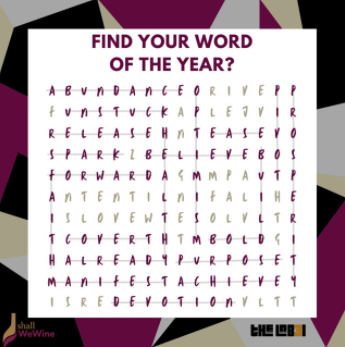At the beginning of the year, I asked Shall We Wine Members to choose a theme song and word for 2021. We meet on NYE and shared our intentions for the year, our words and our song.

Check out this playlist created for us by The Lab3L, a Chicago-based DJ & Production Duo. Let these words and tunes set the tone for all of the amazingness that is yet to come! Playlist is available for streaming here:

 table div table+table+table+table+table+table+table+table+table+table+table+table+table+table+table+table+table+table+table+table+table+table+table+table+table+table+table+table+table+table+table+table div table{width:100%;padding:0}table div table+table+table+table+table+table+table+table+table+table+table+table+table+table+table+table+table+table+table+table+table+table+table+table+table+table+table+table+table+table+table+table div table img{width:96.23%;padding:0;float:none}table div table+table+table+table+table+table+table+table+table+table+table+table+table+table+table+table+table+table+table+table+table+table+table+table+table+table+table+table+table+table+table+table div table td{width:100%;padding:0 1.88% 18px}/* styles */## ON-THE-GO: THE WINE SPRITZER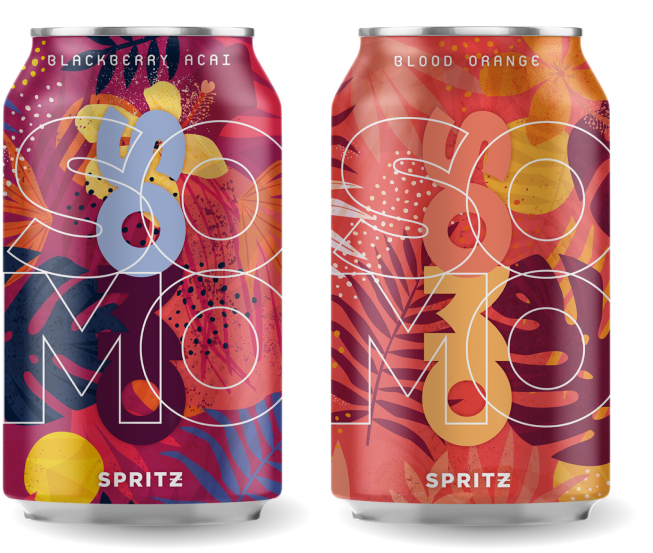The peak of seasonal sunshine has us thinking of the glorious warmth of spring and summertime!

Have you thought about reaching for a ready-to-go, no fuss, wine spritzer? Similar to the hard seltzer, wine spritzers are refreshing, quick options and tend to be low in alcohol. However, the primary difference is that wine spritzers are wine-based, imparting more flavor. Like a glass of wine with just a touch of fruit fizziness!

Enter SOMO Spritz, a canned wine spritzer made with real fruit. No chemical additives or artificial coloring. The color you see is straight up fruit! 7% ABV.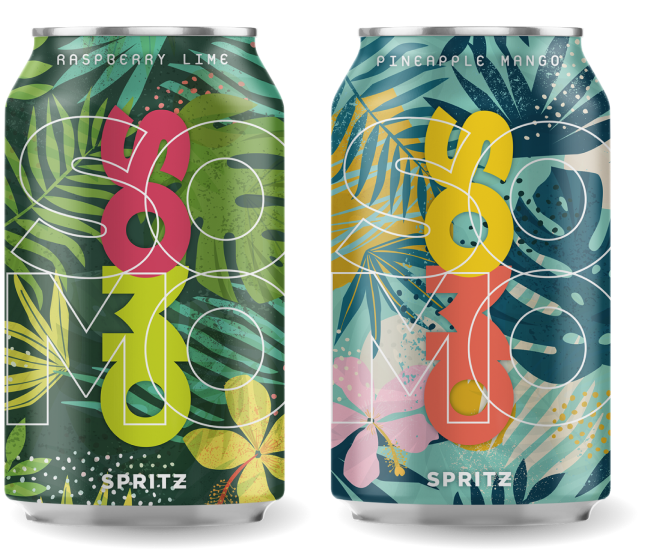Flavor Combos Available:
Pineapple Mango
Raspberry Lime
Blackberry Acai
Blood Orange

Disclosure: Sample cans of SOMO Spritz were received. Samples submitted to Shall We Wine are for review and thoroughly assessed before posting.

 table div table+table+table+table+table+table+table+table+table+table+table+table+table+table+table+table+table+table+table+table+table+table+table+table+table+table+table+table+table+table+table+table+table+table+table+table div table{width:100%;padding:0}table div table+table+table+table+table+table+table+table+table+table+table+table+table+table+table+table+table+table+table+table+table+table+table+table+table+table+table+table+table+table+table+table+table+table+table+table div table img{width:96.23%;padding:0;float:none}table div table+table+table+table+table+table+table+table+table+table+table+table+table+table+table+table+table+table+table+table+table+table+table+table+table+table+table+table+table+table+table+table+table+table+table+table div table td{width:100%;padding:0 1.88% 18px}/* styles */## BLOG CENTRALWe have a blog! Here you will find things like our Wine of the Week and other special wine & spirits topics! Stay in the know and read up on some of the cool things we've been sharing.

 table div table+table+table+table+table+table+table+table+table+table+table+table+table+table+table+table+table+table+table+table+table+table+table+table+table+table+table+table+table+table+table+table+table+table+table+table+table+table+table div table{width:100%;padding:0}table div table+table+table+table+table+table+table+table+table+table+table+table+table+table+table+table+table+table+table+table+table+table+table+table+table+table+table+table+table+table+table+table+table+table+table+table+table+table+table div table img{width:96.23%;padding:0;float:none}table div table+table+table+table+table+table+table+table+table+table+table+table+table+table+table+table+table+table+table+table+table+table+table+table+table+table+table+table+table+table+table+table+table+table+table+table+table+table+table div table td{width:100%;padding:0 1.88% 18px}/* styles */## ENGAGING READS FOR THE MONTHAmerican Wine History has Deep Midwestern Roots
Wine Enthusiast

 table div table+table+table+table+table+table+table+table+table+table+table+table+table+table+table+table+table+table+table+table+table+table+table+table+table+table+table+table+table+table+table+table+table+table+table+table+table+table+table+table+table+table div table{width:100%;padding:0}table div table+table+table+table+table+table+table+table+table+table+table+table+table+table+table+table+table+table+table+table+table+table+table+table+table+table+table+table+table+table+table+table+table+table+table+table+table+table+table+table+table+table div table img{width:96.23%;padding:0;float:none}table div table+table+table+table+table+table+table+table+table+table+table+table+table+table+table+table+table+table+table+table+table+table+table+table+table+table+table+table+table+table+table+table+table+table+table+table+table+table+table+table+table+table div table td{width:100%;padding:0 1.88% 18px}/* styles */## STAY CONNECTED

 /* styles */ To stay in the know with the latest and greatest, make sure to follow us! Links are below.
 table div table+table+table+table+table+table+table+table+table+table+table+table+table+table+table+table+table+table+table+table+table+table+table+table+table+table+table+table+table+table+table+table+table+table+table+table+table+table+table+table+table+table+table+table+table div table{width:100%;padding:0}table div table+table+table+table+table+table+table+table+table+table+table+table+table+table+table+table+table+table+table+table+table+table+table+table+table+table+table+table+table+table+table+table+table+table+table+table+table+table+table+table+table+table+table+table+table div table table{padding:0;float:left!important;width:26.059%!important}table div table+table+table+table+table+table+table+table+table+table+table+table+table+table+table+table+table+table+table+table+table+table+table+table+table+table+table+table+table+table+table+table+table+table+table+table+table+table+table+table+table+table+table+table+table div table td{padding-left:29px;padding-right:29px}table div table+table+table+table+table+table+table+table+table+table+table+table+table+table+table+table+table+table+table+table+table+table+table+table+table+table+table+table+table+table+table+table+table+table+table+table+table+table+table+table+table+table+table+table+table div table table+table,table div table+table+table+table+table+table+table+table+table+table+table+table+table+table+table+table+table+table+table+table+table+table+table+table+table+table+table+table+table+table+table+table+table+table+table+table+table+table+table+table+table+table+table+table+table div table table+table+table{float:left!important;width:26.059%!important}table div table+table+table+table+table+table+table+table+table+table+table+table+table+table+table+table+table+table+table+table+table+table+table+table+table+table+table+table+table+table+table+table+table+table+table+table+table+table+table+table+table+table+table+table+table div table table td,table div table+table+table+table+table+table+table+table+table+table+table+table+table+table+table+table+table+table+table+table+table+table+table+table+table+table+table+table+table+table+table+table+table+table+table+table+table+table+table+table+table+table+table+table+table div table table+table td,table div table+table+table+table+table+table+table+table+table+table+table+table+table+table+table+table+table+table+table+table+table+table+table+table+table+table+table+table+table+table+table+table+table+table+table+table+table+table+table+table+table+table+table+table+table div table table+table+table td{padding-left:0;padding-right:20px}table div table+table+table+table+table+table+table+table+table+table+table+table+table+table+table+table+table+table+table+table+table+table+table+table+table+table+table+table+table+table+table+table+table+table+table+table+table+table+table+table+table+table+table+table+table div table table+table+table+table{float:left!important;width:21.822999999999993%!important}table div table+table+table+table+table+table+table+table+table+table+table+table+table+table+table+table+table+table+table+table+table+table+table+table+table+table+table+table+table+table+table+table+table+table+table+table+table+table+table+table+table+table+table+table+table div table table+table+table+table td{padding-left:0;padding-right:0}/* styles *//* styles */ Learn, Drink, Repeat! The Shall We Wine Team
 Like   Tweet   in